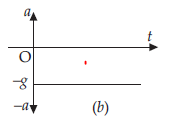# Motion Graphs Worksheet with Solutions

In this page we have Motion Graphs Worksheet with Answers . Hope you like them and do not forget to like , social share and comment at the end of the page.

## Multiple Choice questions

Question 1
A ball is dropped vertically from a height h above the ground .It hits the ground and bounces up vertically to a height h/2.Neglecting subsequent motion and air resistance ,its velocity v varies with the height h as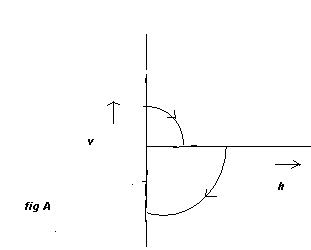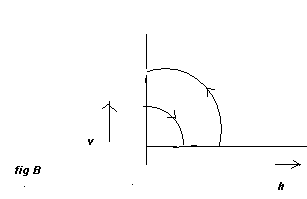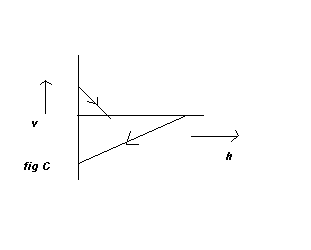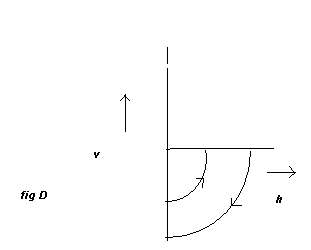Solution
Before hitting the ground ,the velocity v is given by
$v^2=2gh$
Which is a Quadratic equation and hence parabolic path.

Downward direction means negative velocity ,
After collision ,the velocity becomes positive and velocity decreases
Further initial upward will be given by
$v_1^2=2g( \frac {h}{2})=gh$

Therefore
$v=v_1 \sqrt {2}$
As the direction is reversed and speed is decreased and it becomes zero at height h/2
Question 2
The displacement -time graph of a moving particle is shown below.The instantaneous velocity of the particle is negative at the point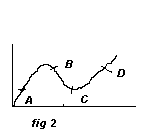(a) C
(b) D
(c) E
(d) B
Solution
The instantaneous velocity is given by the slope of the displacement time graph.
Since slope is negative at point B, instantaneous velocity is negative at B
Hence (d) is correct

Question 3
The velocity -time graph of a moving particle is shown below.Total displacement of the particle during the time interval when there is non zero acceleration and retardation is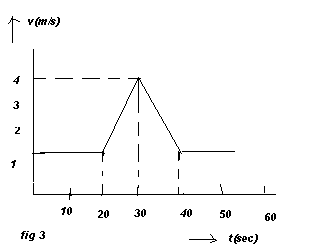(a) 60 m
(b) 40 m
(c) 50 m
(d) 30 m
Solution
Acceleration is given by the slope of the velocity time graph
Non zero acceleration happened in the time interval 20 to 40 sec as the slope of the graph is non zero in that time interval

Now displacement in that interval is given by the area enclosed the v-t curve during that interval

So area=(1/2)20*3 +20*1=50 m
Hence (c) is correct

Question 4
Figure below shows the displacement -time graph of two particles.Mark the correct statement about their relative velocity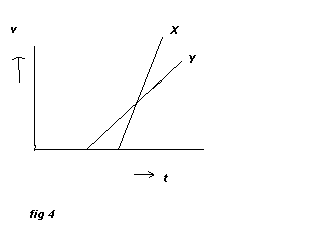(a) It first increases and then decreases
(b) It is a non zero constant
(c) it is zero
(d) none of the above
Solution
The instantaneous velocity is given by the slope of the displacement time graph.
As slope of both the particle displacement time graph is constant.That means there individual velocities are constant.
So relative velocity is also constants
so it is a non zero constant as they have different velocity
Hence (b) is correct

Four position -time graph are shown below.
(a)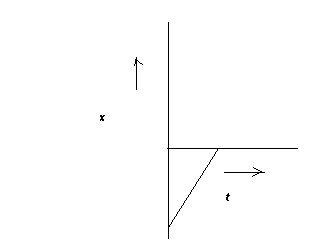(b)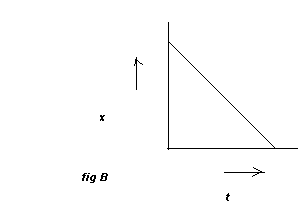(c)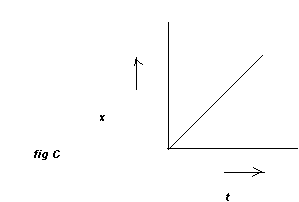(d)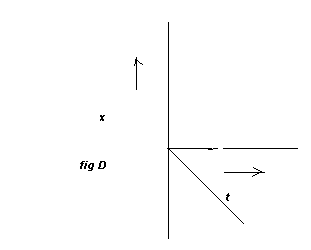Question 5
What all graph shows motion with positive velocities
(a) a and c only
(b) all the four
(c) b and D only
(d) b only
Solution
The instantaneous velocity is given by the slope of the displacement time graph.
Since slope is positive in graph a and c.Positive velocity is there in a and c curve
Hence (a) and (c) are correct

Question 6
What all graph shows motion with negative velocities
(a) a and b only
(b) all the four
(c) a and c only
(d) c only
Solution
The instantaneous velocity is given by the slope of the displacement time graph.
Since slope is negative in graph b and d.Negative velocity is there in b and d curve
Hence (b) and (d) are correct

## Multiple Choice questions with more than one answers

Question 7
which of the following graph correctly represents velocity-time relationships for a particle released from rest to fall under gravity
(a)(b)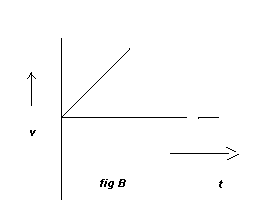(c)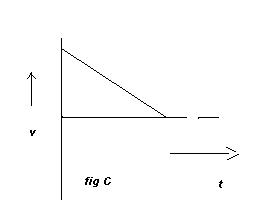(d)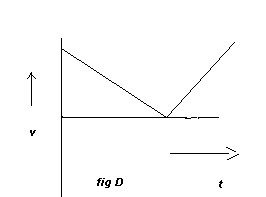Solution
The velocity will increase with time so b is the correct answer
Hence (b) is correct

Question 8
The v-x graph of a particle moving along a straight line is shown below.Which of the below graph shows a-x graph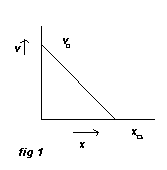(a)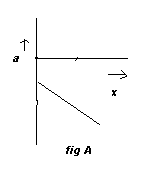(b)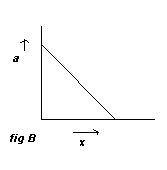(c)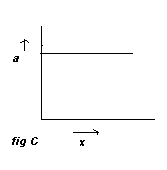(d)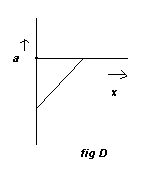Solution
The equation for the given graph is
$v=-(\frac {v_0}{x_0})x + v_0$ ---(1)
Differentiating both sides we get
$\frac {dv}{dx}=- \frac {v_0}{x_0}$ ---(2)
Now
$a=v(\frac {dv}{dx})$
or
$a=(-\frac {v_0}{x_0})[-(\frac {v_0}{x_0})x + v_0 ]$
or
$a=mx+c$
where $m= \frac {v_0^2}{x_0^2}$
and $c=- \frac {v_0^2}{x_0}$

So that means slope is positive and intercept is negative
So (d) is correct

Question 9
Among the four graphs given below, there is only one graph for which Average velocity over the time interval (0, T) can vanish for a suitably chosen T. Which one is it?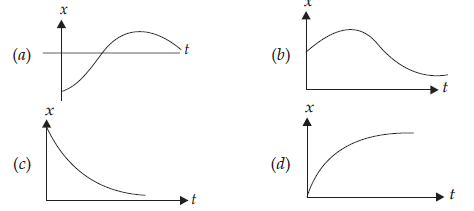Solution
We know that Average velocity is defined as
$\text{average velocity} =\frac {displacement}{time}$
Average velocity of body will be zero when displacement is zero any time interval-T in x-t graph. Now displacement is given by the line joining in the graph.Now initial point given as per question t=0.So We have to find the line which start at t=0 in the graph and is parallel to x axis and intersect at any point on the graph If we check all the graph, we can see that option(b) also satisfies that condition.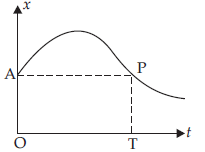Question 10
The variation of quantity A with quantity B, plotted in figure. Describe the motion of a particle in straight line.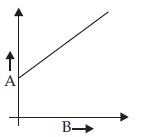(a) Quantity B may represent time.
(b) Quantity A is velocity if motion is uniform.
(c) Quantity A is displacement if motion is uniform.
(d) Quantity A is velocity if motion is uniformly accelerated
Solution
Option (a, c, d) are correct
Explanation: If B represents time and A represents velocity then graph become (v-t). v-t graph is straight line so it is uniformly accelerated motion, so motion is not uniform. Verifies option (a), (d).
If B represents time and A represents displacement, then graph become (s-t) graph. Here s-t graph is straight line which represents uniform motion, so verifies the option (c).

## Match the column

Question 11
For the graph belowMatch the column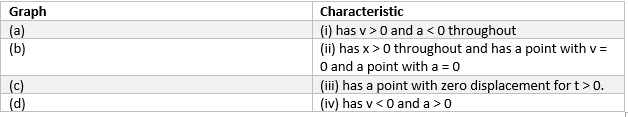Solution
Graph (a) matches with (iii) has a point with zero displacement for t > 0
Reason:it is the only graph where x=0
Graph (b) matches with (ii) has x > 0 throughout and has a point with v = 0 and a point with a=0
Reason: x> 0 through and has a point with velocity v=0 i.e slope zero
Graph (c) matches (iv) has v < 0 and a > 0.
Reason: v < 0 (slope is negative)
Graph (d) matches with (i) has v > 0 and a < 0 throughout
Reason: v > 0 ( slope is positive through out ) and a < 0 as slope is decreasing

Question 12
Match the Column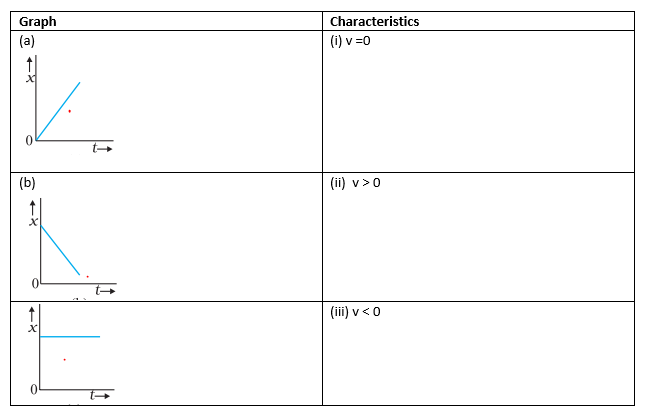Solution
Graph (a) matches with (ii) v > 0
Reason : x is increasing in positive direction
Graph (b) matches with (iii) v < 0
Reason : x is decreasing, so displacement is negative.Hence v < 0
Graph (c) matches with (i) v = 0
Reason : x is constant. So there is no displacement. hence v=0

Question 13
Match the Column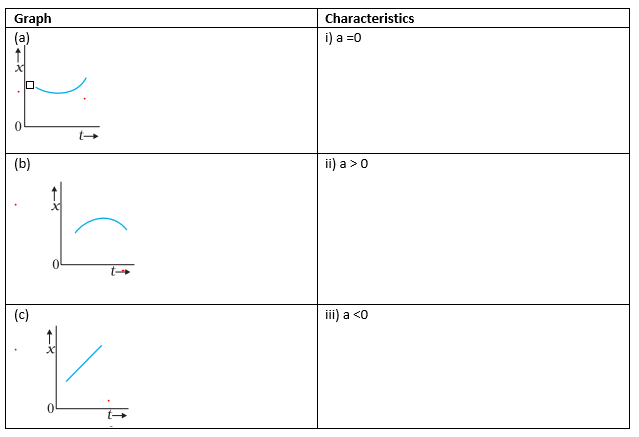Solution
Graph (a) matches with (ii) a > 0
Graph (b) matches with (iii) a < 0
Graph (c) matches with (i) a = 0

Question 14
Match the Column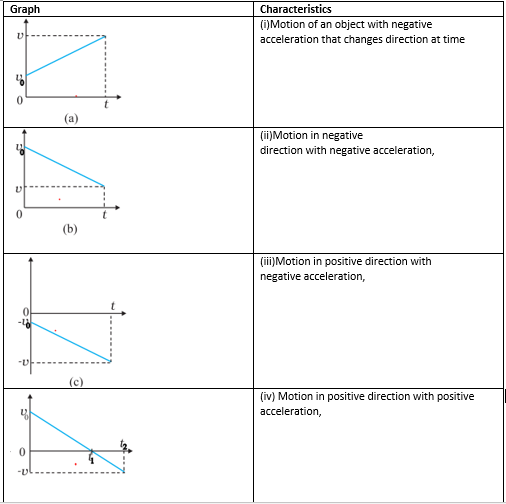Solution
Graph (a) matches with (iv) Motion in positive direction with positive acceleration
Graph (b) matches with (iii) Motion in positive direction with negative acceleration
Graph (c) matches with (ii) Motion in negative direction with negative acceleration
Graph (d) matches with (i) Motion of an object with negative acceleration that changes direction at time

## Subjective Based Questions

Question 15
A ball is dropped and its displacement-time graph is as shown below (displacement x is from ground and all quantities are +ve upwards).
(a) Plot qualitatively velocity vs time graph.
(b) Plot qualitatively acceleration vs time graph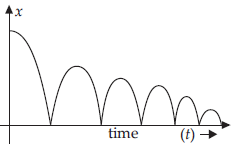Solution
a. For velocity (v-t graph)
While going downward, Velocity of body increases till the x becomes zero then velocity becomes in opposite direction and velocity (slope of x-t graph) decreases to zero till it reaches maximum value of x but smaller than earlier.So velocity moves between negative and positive with magnitude decreasing with every bounce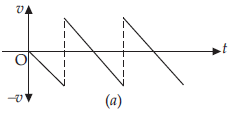b.For acceleration(a-t graph)
When velocity increases and body reaches towards x = 0 acceleration is in downward direction. When body moves upward i.e., x > 0 then velocity decreases so direction of 'a' is again downward.
So a=-g through out the motion